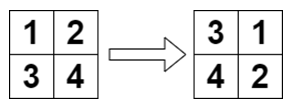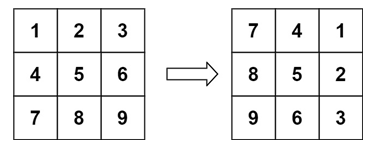Rate this post

[toc]

Are you terrified of being asked this question in an interview? Don’t worry! You are not alone. Many people found it intimidating. Unfortunately, the probability of seeing it at least once is quite high if you are undergoing a lot of interviews. Many interviewee’s have claimed that they saw it multiple times! Hence, this is one of those few questions which require a lot of practicing to ensure you can confidently code it and explain it without thinking too much.

## Problem Formulation

You are given an n x n 2D matrix representing an image. Rotate the image by 90 degrees (clockwise). You need to do this in place.

⚠️ Constraints:

• `matrix.length == n`
• `matrix[i].length == n`
• `1 <= n <= 20`
• `-1000 <= matrix[i][j] <= 1000`

? Challenge: Try not to allocate another 2D matrix and do the rotation.

## ?Examples

Let’s have a look at some examples to improve our understanding of this problem.

```✏️ Example 1

Input: matrix = [[1,2],[3,4]]
Output: [[3,1],[4,2]]
Explanation:✏️ Example 2
Input: matrix = [[1,2,3],[4,5,6],[7,8,9]]
Output: [[7,4,1],[8,5,2],[9,6,3]]
Explanation:✏️ Example 3
Input: matrix = []
Output: []
Explanation: This is an edge case.```

Now let us dive into the solutions to the given problem.

## ?️Solution 1: Using Extra Space

Approach

The approach can be best understood with the help of an example. Hence, let us consider the following matrix to understand the demonstration.

Now if you notice carefully, you will find that if you reverse the column ‘i’ then it will correspond to the new row in ‘i’ in resultant matrix. For example:

• column 0 in original matrix in reverse order is `7 4 1` which corresponds to row 0 in resultant matrix.
• column 1 in original matrix in reverse order is `8 5 2` which corresponds to row 1 in resultant matrix.
• column 2 in original matrix in reverse order is `9 6 3` which corresponds to row 2 in resultant matrix.

You can simply implement this approach and keep storing the result in another 2 D array. Now, let’s visualize where the elements need to end up in the resultant array in each iteration.

Now, it is time to dive into the code:

```def rotate_matrix(matrix):
n = len(matrix)
k = [[0 for i in range(n)] for j in range(n)]

for i in range(n):
for j in range(n):
k[j][n - i - 1] = matrix[i][j]
return k```

Let’s execute the test cases on the code.

Complexity Analysis: The runtime complexity of this approach is O(M) where `M` denotes the number of cells in the given matrix.

Discussion: This approach is quite straightforward. However, it does not fully satisfy the purpose of this question. It was mentioned that we have to rotate the matrix “in place” (no extra space allowed.) and we did not satisfy this condition as we stored the output in a different matrix . This approach consumes an additional space of O(n2) where `n = number of rows in 2D array`. Hence, is there a way to avoid storing the output in a different matrix to reach the solution?

## ?️Solution 2: In Place Rotation

Approach: To ensure rotating the matrix without consumption of extra space, you have to move 4 elements within the matrix simultaneously in groups of four. To visualize this approach let’s consider the above given matrix once again.

Here, the following operation needs to occur in the first iteration:

• 7 needs to end up in 1’s position.
• If 7 goes to 1’s position, then we need to check where 1 needs to go otherwise value 1 will be lost. Thus, 1 needs to go to 3’s position.
• 3 needs to go to 9’s position.
• 9 needs to go to 7’s position.
• We already have placed 7 in 1’s position.

Similar adjustments have to be made in each iteration to maintain constant memory usage. The question is, how do we achieve this feat? You can do this in two steps –

Step 1: Transpose the matrix.

?️ Transpose of a matrix is obtained by transforming the columns to rows and the rows to columns. Thus, transpose of a matrix A[row][column] is obtained by transforming A[row][column] to A[column][row].

Code to transpose the matrix:

```for row in range(len(matrix)):
for col in range(row, len(matrix)):
matrix[row][col], matrix[col][row] = matrix[col][row], matrix[row][col]```

Step 2: Reverse the rows of the transposed matrix.

Once you have transposed the matrix, you just have to reverse the rows of the transpose matrix to derive the output matrix. The following code does exactly that.

```n = len(matrix)
for i in range(n // 2):
for j in range(n):
matrix[j][i], matrix[j][n - 1 - i] = matrix[j][n - 1 - i], matrix[j][i]```

Let’s visualize what happens to the matrix in each iteration.

Now, all that’s left to be done is to club the two steps together. Hence, let’s look at the complete solution.

```def rotate_matrix(matrix):
# transpose the matrix
for row in range(len(matrix)):
for col in range(row, len(matrix)):
matrix[row][col], matrix[col][row] = matrix[col][row], matrix[row][col]
n = len(matrix)
# swap columns moving inwards from outwards
for i in range(n // 2):
for j in range(n):
matrix[j][i], matrix[j][n - 1 - i] = matrix[j][n - 1 - i], matrix[j][i]
return matrix```

Time to execute the test cases on our code and check its efficiency.

```Example 1
matrix = [[1, 2], [3, 4]]
print(rotate_matrix(matrix))
# [[3, 1], [4, 2]]

Example 2
matrix = [[1, 2, 3], [4, 5, 6], [7, 8, 9]]
print(rotate_matrix(matrix))
# [[7, 4, 1], [8, 5, 2], [9, 6, 3]]

Example 3
matrix = []
print(rotate_matrix(matrix))
# []```

That is exactly what we expected and our approach passed all the test cases.

Complexity Analysis

Let M be the number of cells in the given matrix.

• Time complexity
•  Transposing the matrix has a runtime complexity of O(M) as we’re moving the value of each cell once.
• Reversing each row also has a runtime complexity of O(M) because once again we’re moving the value of each cell once.
• Hence the Total Time Complexity of our code is O(M)
• Space complexity: Since we are not using any other additional data structures, the space complexity in this case is O(1).

## Conclusion

I hope you enjoyed this coding interview question. Please stay tuned and subscribe for more interesting coding problems.